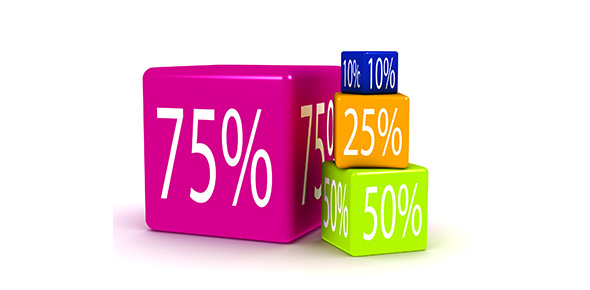# Test Your Comprehension And Understanding Of Percentages

15 Questions | Attempts: 3903SettingsThe percentage quiz 12 is designed to test your comprehension and understanding of percentages. This is mostly by understanding statements and finding the percentages required. All the best as you test and advance your ability.

• 1.
In 1990, Mary's height was 61 cm. In 2000, she grew to 130 cm. Find the percent increase in Mary's height.
• A.

113.12%

• B.

53.07%

• C.

146%

• 2.
What percent of 20 is 30?
• A.

100%

• B.

150%

• C.

66.67%

• 3.
What is 35% of 80?
• A.

28

• B.

43.75

• C.

228

• 4.
45% of what is 9?
• A.

4.05

• B.

20

• C.

5

• 5.
Suppose a certain item used to sell for 75 cents a pound, you see that it's been marked up to 81 cents a pound. What is the percent increase?
• A.

8%

• B.

10%

• C.

18%

• 6.
A golf shop pays its wholesaler \$40 for a certain club, and then sells it to a golfer for\$75. What is the percent change?
• A.

46.66%

• B.

51%

• C.

87.5%

• 7.
An item originally priced at \$55 is marked 25% off. What is the sale price?
• A.

\$53.35

• B.

\$12.10

• C.

\$41.25

• 8.
An item that regularly sells for \$425 is marked down to \$318.75. What is the percent change?
• A.

175%

• B.

33.33%

• C.

25%

• 9.
Your friend diets and goes from 125 pounds to 110 pounds. What was her percentage weight loss?
• A.

12%

• B.

15%

• C.

8%

• 10.
Jimmy got a raise from \$6.00 an hour to \$8.00 an hour. This is what percent change?
• A.

33.33%

• B.

25%

• C.

2.33%

• 11.
Sandra bought a stock for \$30, and she sold it for \$36.  She made a profit of what percent?
• A.

30%

• B.

16.67%

• C.

20%

• 12.
A cookbook was reduced from \$24.50 to \$17.95.  This was a reduction of what percent?
• A.

74%

• B.

36.4%

• C.

26.7%

• 13.
If your salary rises from \$33,000 to \$45,000, what is the percent change?
• A.

36.3%

• B.

26.7%

• C.

50%

• 14.
The original price of a shirt was \$20. It was decreased to \$15 . What is the percent decrease of the price of this shirt?
• A.

30%

• B.

25%

• C.

52%

• 15.
The price of a DVD player changed from \$120 to \$100. Then later the price decreased again from \$100 to \$80. Which of the two decreases was larger in percentage term?
• A.

The first decrease

• B.

The second decrease

## Related TopicsBack to top
×

Wait!
Here's an interesting quiz for you.